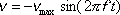For an object undergoing simple harmonic motion, this equation gives the velocity of the object as a function of time.

v is the velocity of the object
vmax is a constant.  It is the maximum velocity of the object.
f is the frequency of oscillations.
t is the time.

Note: vmax does depend on the frequency and amplitude of the oscillations (see "vmax = 2 pi f" on formula sheet).  We call vmax  a constant because once the object undergoing simple harmonic motion is set into motion, vmax does not change.

Tip: It is typically easier to find the velocity of the object at a specified position using conservation of energy than it is using this equation.

Pitfall Avoidance Note: The v in this equation is not wave velocity. This v is the velocity of an object undergoing simple harmonic motion. To be sure, although this v was introduced in the simple harmonic motion part of the physics course, not the wave part, it does have something to do with wave motion. Consider a vertical wave in a horizontal string. Each point on the string undergoes simple harmonic motion. Each point goes up and down while the wave itself travels along the length of the string. Pick any point along the length of the string. The simple harmonic motion equations apply to that point. The frequency of the wave and the amplitude of the wave are the frequency of the oscillations and the amplitude of oscillations of that point. But the speed of that point is not the speed of the wave. How fast that point is going upward, as a function of time, is indeed given by the equation on this page. The speed of the wave however, how fast the pattern moves forward along the length of the string, is given by the equation v = lambda / T where lambda is the wavelength of the wave, a characteristic not possessed by a simple harmonic oscillator.